﻿ VANET云环境下基于人工神经网络的车辆任务卸载策略文章快速检索 高级检索
 重庆邮电大学学报(自然科学版)2020, Vol. 32Issue (3): 336-344  DOI: 10.3979/j.issn.1673-825X.2020.03.0020

### 引用本文LIANG Yucheng, CAO Bin. Artificial neural network methods for task offloading in VANET cloud[J]. Journal of Chongqing University of Posts and Telecommunications (Natural Science Edition), 2020, 32(3): 336-344.   DOI: 10.3979/j.issn.1673-825X.2020.03.002..

### 文章历史

VANET云环境下基于人工神经网络的车辆任务卸载策略

1. 重庆邮电大学 移动通信技术重庆市重点实验室, 重庆 400065;
2. 北京邮电大学 网络与交换技术国家重点实验室, 北京 100876

LIANG Yucheng1, CAO Bin21. Chongqing Key Lab of Mobile Communications Technology, Chongqing University of Posts and Telecommunications, Chongqing 400065, P. R. China;
2. State Key Laboratory of Network and Switching Technology, Beijing University of Posts and Telecommunications, Beijing 100876, P. R. China
0 引言

VANET云其独特之处在于云服务器可以是具有丰富资源或者较好互联网接入能力的车辆，资源受限车辆希望租用这些车辆的空闲资源。为实现这一目标，需求车辆需要寻找这些资源空闲车辆，了解其资源状态，并与他们进行通信和资源请求。但是，由于车辆的高移动性，会导致卸载过程中丢失与目标车辆的通信，造成任务卸载失败。此外，共享自身计算资源意味着能耗增加，需要考虑如何激励车辆共享自身计算资源，在最大化自身收益的同时又能满足需求车辆的资源需求。针对以上问题，我们采用广泛应用于道路云环境中的VANET云与软件定义网络(software defined network, SDN)相结合的方法[7-9]，在路侧单元(road side unit, RSU)部署SDN控制器，通过将车辆通信的控制面与数据面分离，实现网络的灵活控制，使网络作为管道变得更加智能。为了实现车辆与RSU以及车辆间进行通信，每个车辆的车载单元(on board unit, OBU)同时配备有蜂窝网络接口和IEEE 802.11p网络接口，车辆通过蜂窝网络向SDN控制器持续发送自身相关信息(包括车辆的位置、速度及通过信标帧收到的周围车辆的ID等)，其系统架构如图 1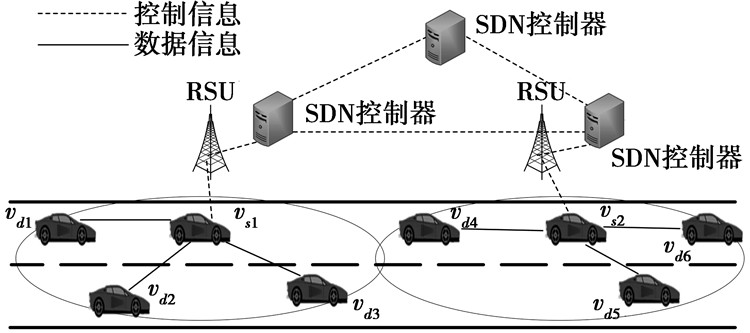图 1 VANET云与SDN结合的智能交通系统架构 Fig.1 System architecture of intelligent traffic system based on VANET cloud and SDN

1 相关工作

2 系统模型

2.1 源车辆卸载效用

 ${U_s} = {u_s} - {C_s}$ (1)

(1) 式中，Us主要包括卸载收益us和卸载开销Cs。令车辆vs需要卸载的总任务量为Wsus表示需求车辆vs将任务Ws卸载至周围所有可用车辆vd可以获得的总卸载收益。

 ${W_s} = \sum\limits_{d = 1}^N {{W_{sd}}}$ (2)

(2) 式中，Wsd表示直接通过V2V链路卸载至周围车辆vd的任务量。当s=d时表示这部分任务在本地处理。

 ${u_s} = \theta \sum\limits_{d = 1}^N {{P_{sd}}} {\rm{lg}}(1 + {W_{sd}})$ (3)

(3) 式中：θ为任务卸载收益权重参数，且其值大于零；Psdvs对应的vd可提供的最大处理速率。

Cs表示车辆vs将任务Ws完全卸载需要的开销，其主要由传输开销Cs, t和本地计算开销Cs, p组成，即Cs=Cs, t+Cs, p。为了简化问题，将V2V链路的传输带宽设定为固定值Bsd。将传输开销定义为

 ${C_{s, t}} = \gamma \sum\limits_{d = 1}^N {{W_{sd}}}$ (4)

(4) 式中，γ为通过V2V链路传输1 Mbit需要的单位传输开销。

2.2 优化模型

 ${{\rm{max}}{\kern 1pt} {\kern 1pt} {\kern 1pt} {\kern 1pt} {\kern 1pt} {\kern 1pt} {\kern 1pt} {\kern 1pt} {\kern 1pt} {\kern 1pt} {U_s}}$ (5)
 ${{\rm{ s}}{\rm{.t}}{\rm{. }}{\kern 1pt} {\kern 1pt} {\kern 1pt} {\kern 1pt} {\kern 1pt} {\kern 1pt} {\kern 1pt} {\kern 1pt} \sum\limits_{s = 1}^M {{P_{sd}}} \le {P_d}, \forall d}$ (6)
 ${\sum\limits_{d = 1}^N {{W_{sd}}} \le {W_s}, \forall s}$ (7)
 ${\frac{{2{W_{sd}}}}{{{B_{sd}}}} + \frac{{{W_{sd}}}}{{{P_{sd}}}} \le T_{sd}^{{\rm{ pre }}}, \forall s, d}$ (8)
 ${{P_{sd}} \ge 0, \forall s, d}$ (9)
 ${{W_{sd}} \ge 0, \forall s, d}$ (10)

(6) 式表示源车辆vs向一辆目标车辆vd购买的计算速率之和不大于其剩余计算速率；(7)式表示需求车辆vs卸载的总任务量不大于其需求卸载任务量；(8)式表示通过V2V链路进行任务卸载的理论计算时间不大于V2V链路的预测连接时间。

3 车辆移动性预测 3.1 Nagel-Shreckenberg车辆交通模型

1) 加速。如果车辆的速度v低于vmax并且观察到距离前方的下一辆车距离大于v+1，则车速由v变为v+1。

2) 减速。如果车辆行驶在位置i处观察到前方下一辆车位于位置i+j(jv)，则将其速度v降低到j－1。

3) 随机减速。当车速不为0时，以概率p速度由v降低到v－1。

4) 汽车移动。每辆车单位时间向前移动v个元胞。

5) 车辆变道。如果车辆观察到与前方车辆距离大于v+1，此时车辆存在一定的概率进行变道行驶，其中，向左的概率为0.8，向右的概率为0.5。当车辆距离前方车辆距离小于安全距离时，车辆会观察左右平行方向是否存在行驶车辆，如果没有则优先向左侧进行变道，反之向右变道。当车辆与前方车辆距离小于安全距离且左右平行车道都被其他车辆占用，则进行减速运行。

3.2 基于神经网络的连接时间预测

1) 真实连接时间。车辆vsvd在Nagel-Shreckenberg模型中的实际连接时间(预测模型的目标输出)。

2) 车辆位置。车辆vsvd的坐标；该路段中vsvd所在RSU关联的其他车辆坐标。

3) 车辆速度。通过观察实时获取的该路段与车辆vsvd所在RSU关联的所有车辆速度。

3.3 算法时间复杂度分析

4 基于买卖博弈的资源分配策略 4.1 有效卸载速率

 $T_{sd}^{{\rm{ct}}} = \left\{ {\begin{array}{*{20}{l}} {\frac{{{W_{sd}}}}{{{B_{sd}}}} + \frac{{{W_{sd}}}}{{{P_{sd}}}} + \frac{{{W_{sd}}}}{{{B_{ds}}}}, }&{s \ne d, \forall s, \forall d}\\ {\frac{{{W_{ss}}}}{{{P_{ss}}}}, }&{s = d, \forall s, \forall d} \end{array}} \right.$ (11)

(11) 式中，Bsd为源车辆和目标车辆间的传输速率。为了简化问题，本文将传输速率Bsd设置为定值，而不同距离下的传输速率变化对任务卸载的影响，将作为我们未来工作的重点。当在本地处理时，Bsd可看做无穷大。因此, 任务的有效卸载速率为

 ${{R_{sd}} = \frac{{{B_{sd}}{P_{sd}}}}{{2{P_{sd}} + {B_{sd}}}}}$ (12)
 ${{\rm{s}}{\rm{.t}}{\rm{.}}{\kern 1pt} {\kern 1pt} {\kern 1pt} {\kern 1pt} {\kern 1pt} {\kern 1pt} {\kern 1pt} {\kern 1pt} {\kern 1pt} {\kern 1pt} {\kern 1pt} {\kern 1pt} {\kern 1pt} {\kern 1pt} {\kern 1pt} {\kern 1pt} \sum\limits_{s = 1}^M {{P_{sd}}} \le {P_d}, \forall j}$ (13)

(13) 式中，Pd为卸载目标可以提供的的最大计算速率。由(12)式可知，有效卸载速率是关于Pij的凸函数。上文提到目标车辆不会无偿共享自身计算资源。为解决这一问题，设计了一种基于买卖博弈的有偿资源分配算法，以激励目标车辆共享自身的空闲资源，参与任务卸载。该算法将需求车辆vs定义为买方，计算资源空余的目标车辆vd定义为卖方，通过建模分析，利用买卖博弈算法获得vd可以提供的最优计算速率与vs可以给出的最优单位计算速率价格。

4.2 买方分析

 ${{G_{{b_{sd}}}} = {\lambda _{sd}}{R_{sd}} - {\alpha _{sd}}{P_{sd}}}$ (14)
 ${{\rm{ s}}{\rm{.t}}{\rm{. }}{\kern 1pt} {\kern 1pt} {\kern 1pt} {\kern 1pt} {\kern 1pt} {\kern 1pt} {\kern 1pt} {\kern 1pt} {\kern 1pt} {\kern 1pt} {\kern 1pt} {\kern 1pt} {\kern 1pt} {\kern 1pt} \sum\limits_{s = 1}^M {{P_{sd}}} \le {P_d}, \forall d}$ (15)

(14) 式中：λsd为单位有效卸载速率的收益；αsd为单位计算速率的售价。通过计算可知卖方收益Gbsd是关于Psd的凸函数。因此，买方收益函数会随着购买的计算速率增加而增加，通过观察买方收益函数和计算速率之间的关系，可以获得计算速率的最优解。即当∂Gbsd/∂Psd=0时，可以得到最优计算速率分配值为

 $P_{sd}^* = \sqrt 2 {B_{sd}}{({\alpha _{sd}})^{ - 0.5}} - 2{B_{sd}}$ (16)
4.3 卖方分析

 ${{G_{{s_{sd}}}} = ({\alpha _{sd}} - {c_{sd}}){P_{sd}}}$ (17)
 ${{\rm{s}}{\rm{.t}}{\rm{.}}{\kern 1pt} {\kern 1pt} {\kern 1pt} {\kern 1pt} {\kern 1pt} {\kern 1pt} {\kern 1pt} {\kern 1pt} {\kern 1pt} {\kern 1pt} {\kern 1pt} {\kern 1pt} {\kern 1pt} {\kern 1pt} \sum\limits_{s = 1}^M {{P_{sd}}} \le {P_d}, \forall d}$ (18)

(17) 式中，csd为卖方单位处理速率的成本价格，由于卸载目标自身属性(包括车辆型号、计算能力、是否愿意参与卸载等)不同，不同卖方初始出价csd也不相同。

 $\frac{{\partial {G_{{s_{sd}}}}}}{{\partial {\alpha _{sd}}}} = P_{sd}^* + ({\alpha _{sd}} - {c_{sd}})\frac{{\partial P_{sd}^*}}{{\partial {\alpha _{sd}}}}$ (19)

4.4 价格更新函数

 $\frac{{\partial {G_{{s_{sd}}}}}}{{\partial {\alpha _{sd}}}} = P_{sd}^* + ({\alpha _{sd}} - {c_{sd}})\frac{{\partial P_{sd}^*}}{{\partial {\alpha _{sd}}}} \ge 0$ (20)

 ${\alpha _{sd}}(t + 1) \le I({\alpha _{sd}}(t))$ (21)

(21) 式中：t为迭代次数；I(αsd(t))为价格更新函数，期表达式为

 $I({\alpha _{sd}}(t)) = {c_{sd}} - \frac{{P_{sd}^*}}{{\frac{{\partial P_{sd}^*}}{{\partial {\alpha _{sd}}(t)}}}}$ (22)

1) for每一个卖方d

2) 初始化迭代次数t=0；

3) 获得卖方初始报价α=c

4) 计算买方资源需求P

5) if $\frac{{\partial {G_s}}}{{\partial \alpha }} > 0$

6) 卖方根据(22)式更新价格

7) t=t+1

8) else if t>0

9) α*=α

10) else

11) α*=c;

12) end if

13) if $\frac{{\partial {G_b}}}{{\partial P}} > 0$

14) 买方根据(22)式更新速率需求

15) else if t>0

16) P*=P

17) else

18) P*=P(c)

19) end if

20) end for

5 集中式任务分配方法

 ${w_{sd}} < T_{sd}^{{\rm{ pre }}}{R_{sd}}$ (23)

1) 获取周围可用目标车辆集合{v1, v2, …, vN}；

2) for每个目标车辆d

3) 使用算法1计算Psd*

4) 根据(12)式计算有效卸载速率Rsd

5) 使用神经网络预测链路sd连接时间Tsdpre

6) end for

7) 根据(5)式，s.t.(6)—(10)式计算

 ${\rm{max}}{U_i}, W_{ss}^*, W_{s1}^*, \cdots , W_{sd}^*, \cdots , W_{MN}^*;$

6 仿真与性能分析

6.1 连接时间预测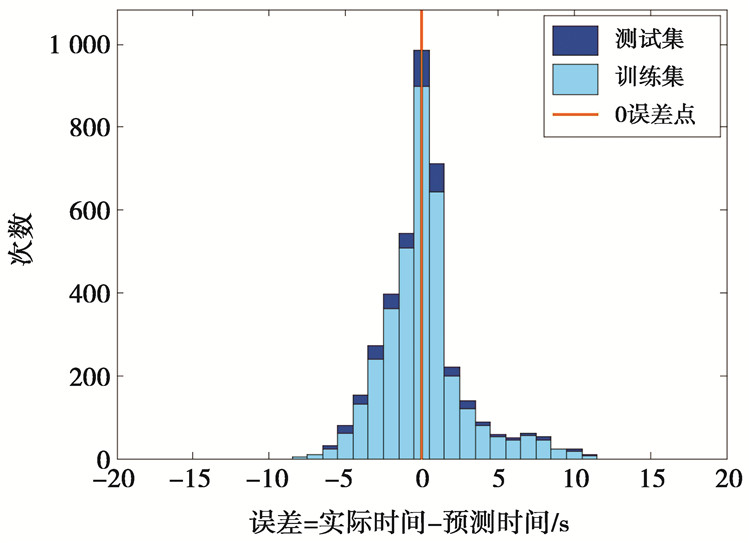图 2 人工神经网络神经网络预测结果 Fig.2 Artificial neural network prediction results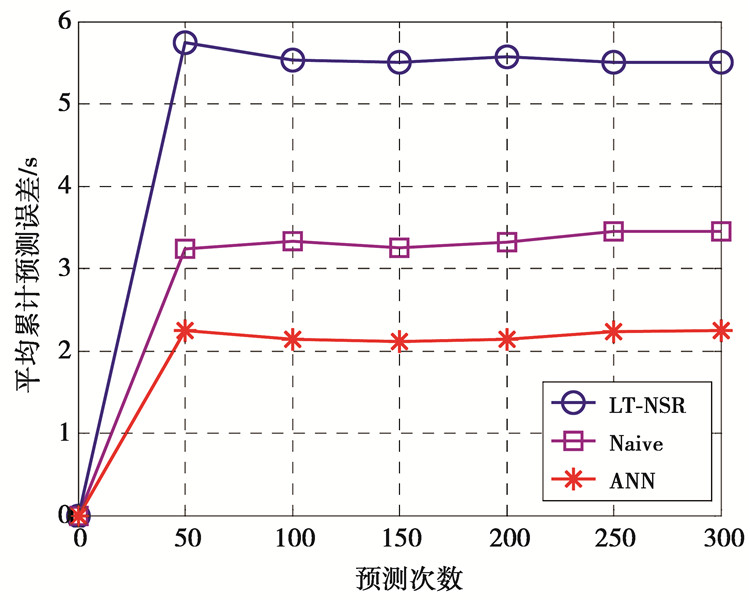图 3 平均预测时间误差对比图 Fig.3 Average prediction time error comparison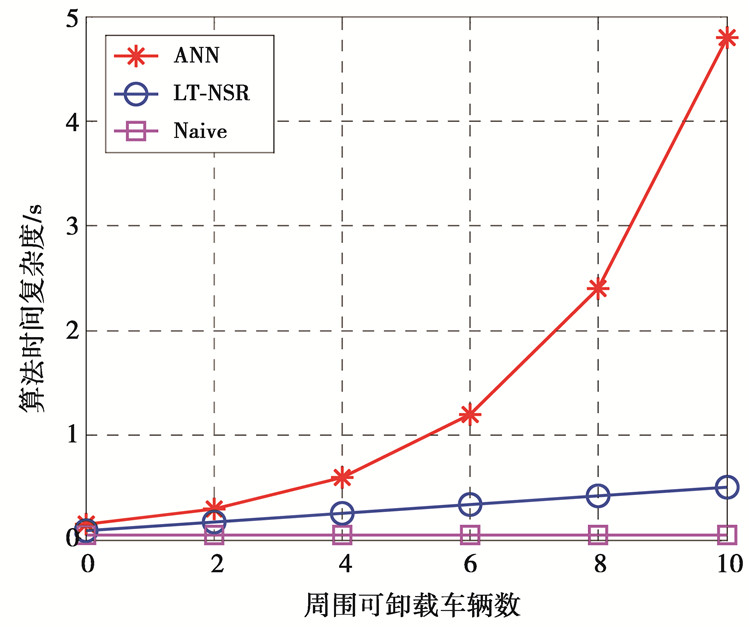图 4 算法时间复杂度对比 Fig.4 Algorithmic complexity comparison
6.2 最优计算速率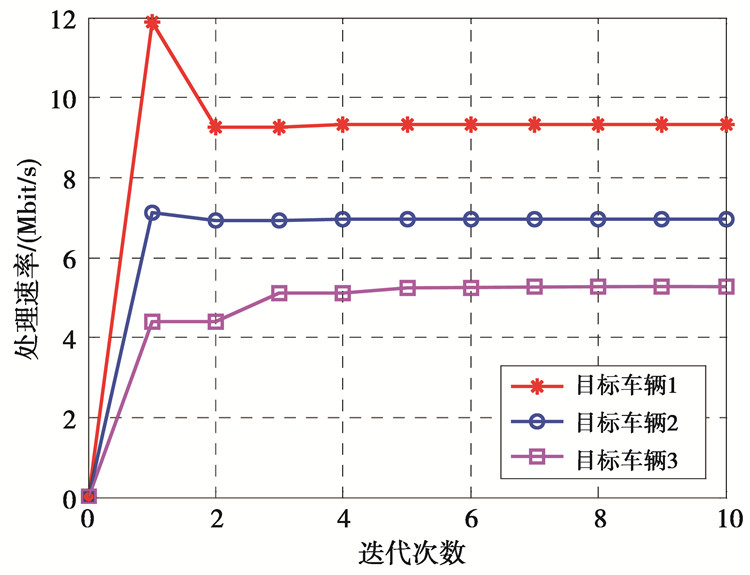图 5 目标车辆处理速率分配更新 Fig.5 Processing rate allocation update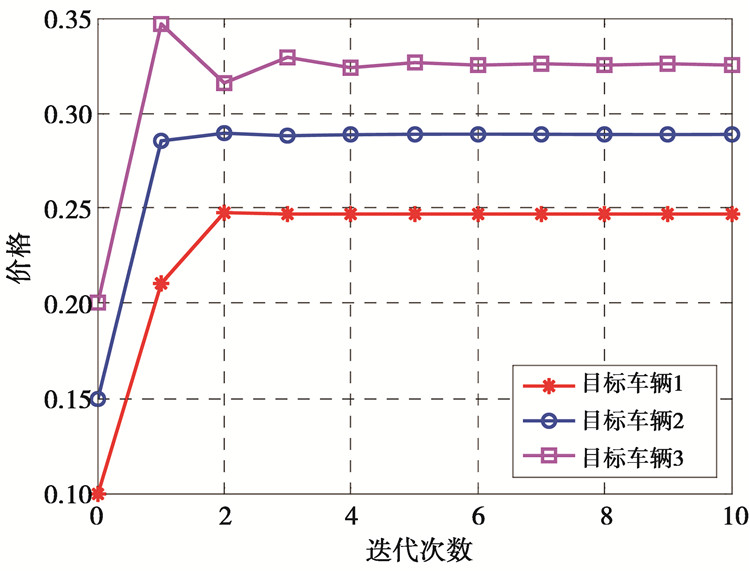图 6 目标车辆价格更新 Fig.6 Price update
6.3 任务分配结果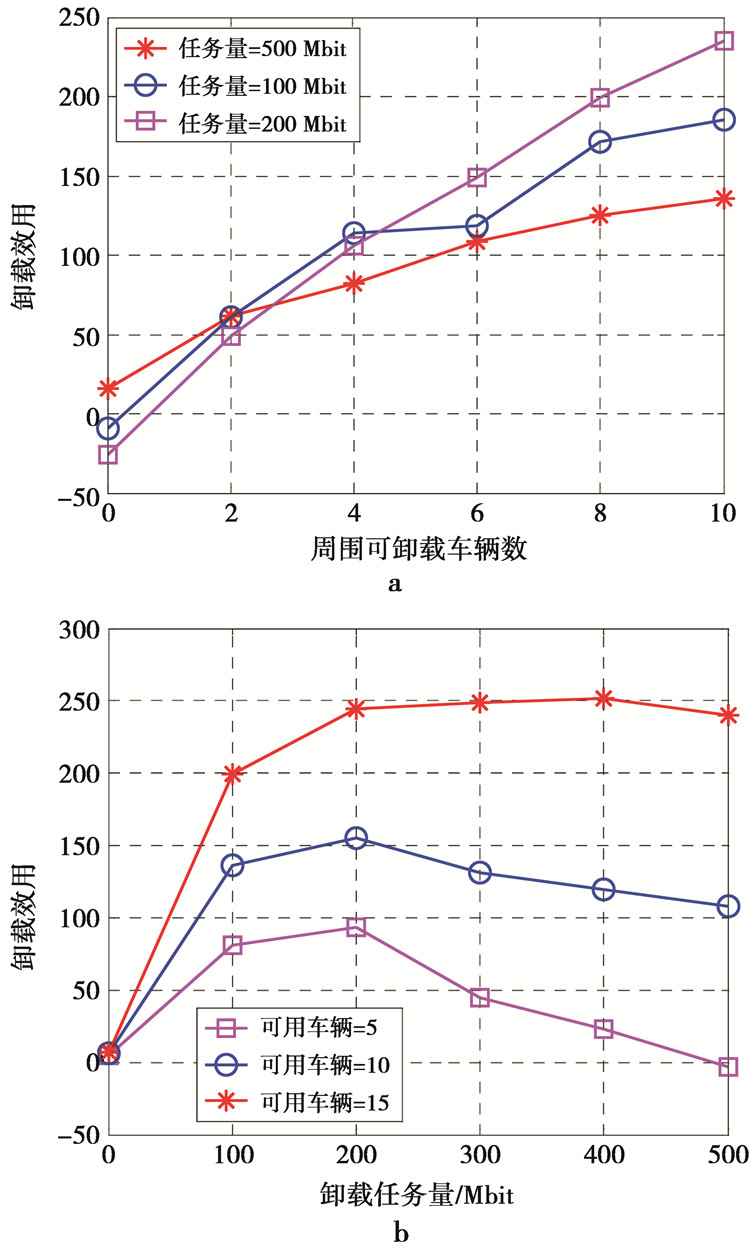图 7 卸载效用对比图 Fig.7 offloading utility comparison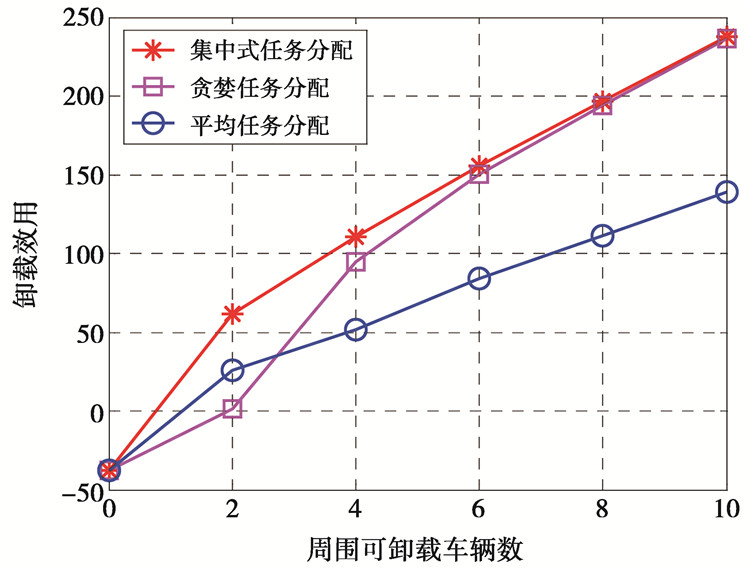图 8 不同任务量与卸载效用关系图对比图 Fig.8 Comparison of different task volumes and offloading utility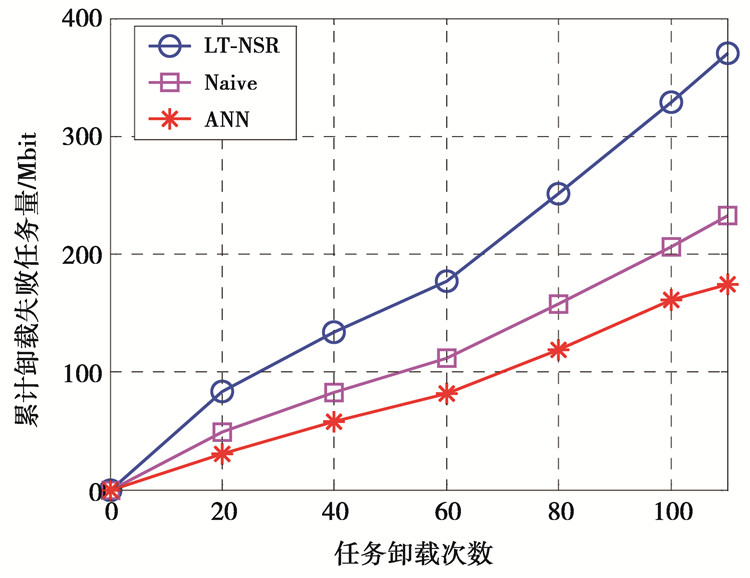图 9 卸载失败任务量对比图 Fig.9 Offloading failure task volume comparison
7 结束语